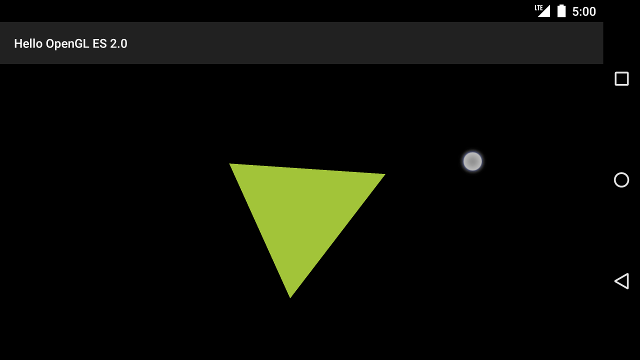# Respond to touch events

Making objects move according to a preset program like the rotating triangle is useful for getting some attention, but what if you want to have users interact with your OpenGL ES graphics? The key to making your OpenGL ES application touch interactive is expanding your implementation of `GLSurfaceView` to override the `onTouchEvent()` to listen for touch events.

This lesson shows you how to listen for touch events to let users rotate an OpenGL ES object.

## Setup a touch listener

In order to make your OpenGL ES application respond to touch events, you must implement the `onTouchEvent()` method in your `GLSurfaceView` class. The example implementation below shows how to listen for `MotionEvent.ACTION_MOVE` events and translate them to an angle of rotation for a shape.

### Kotlin

```private const val TOUCH_SCALE_FACTOR: Float = 180.0f / 320f
...
private var previousX: Float = 0f
private var previousY: Float = 0f

override fun onTouchEvent(e: MotionEvent): Boolean {
// MotionEvent reports input details from the touch screen
// and other input controls. In this case, you are only
// interested in events where the touch position changed.

val x: Float = e.x
val y: Float = e.y

when (e.action) {
MotionEvent.ACTION_MOVE -> {

var dx: Float = x - previousX
var dy: Float = y - previousY

// reverse direction of rotation above the mid-line
if (y > height / 2) {
dx *= -1
}

// reverse direction of rotation to left of the mid-line
if (x < width / 2) {
dy *= -1
}

renderer.angle += (dx + dy) * TOUCH_SCALE_FACTOR
requestRender()
}
}

previousX = x
previousY = y
return true
}
```

### Java

```private final float TOUCH_SCALE_FACTOR = 180.0f / 320;
private float previousX;
private float previousY;

@Override
public boolean onTouchEvent(MotionEvent e) {
// MotionEvent reports input details from the touch screen
// and other input controls. In this case, you are only
// interested in events where the touch position changed.

float x = e.getX();
float y = e.getY();

switch (e.getAction()) {
case MotionEvent.ACTION_MOVE:

float dx = x - previousX;
float dy = y - previousY;

// reverse direction of rotation above the mid-line
if (y > getHeight() / 2) {
dx = dx * -1 ;
}

// reverse direction of rotation to left of the mid-line
if (x < getWidth() / 2) {
dy = dy * -1 ;
}

renderer.setAngle(
renderer.getAngle() +
((dx + dy) * TOUCH_SCALE_FACTOR));
requestRender();
}

previousX = x;
previousY = y;
return true;
}
```

Notice that after calculating the rotation angle, this method calls `requestRender()` to tell the renderer that it is time to render the frame. This approach is the most efficient in this example because the frame does not need to be redrawn unless there is a change in the rotation. However, it does not have any impact on efficiency unless you also request that the renderer only redraw when the data changes using the `setRenderMode()` method, so make sure this line is uncommented in the renderer:

### Kotlin

```class MyGlSurfaceView(context: Context) : GLSurfaceView(context) {

init {
// Render the view only when there is a change in the drawing data
renderMode = GLSurfaceView.RENDERMODE_WHEN_DIRTY
}
}
```

### Java

```public MyGLSurfaceView(Context context) {
...
// Render the view only when there is a change in the drawing data
setRenderMode(GLSurfaceView.RENDERMODE_WHEN_DIRTY);
}
```

## Expose the rotation angle

The example code above requires that you expose the rotation angle through your renderer by adding a public member. Since the renderer code is running on a separate thread from the main user interface thread of your application, you must declare this public variable as `volatile`. Here is the code to declare the variable and expose the getter and setter pair:

### Kotlin

```class MyGLRenderer4 : GLSurfaceView.Renderer {

@Volatile
var angle: Float = 0f
}
```

### Java

```public class MyGLRenderer implements GLSurfaceView.Renderer {
...

public volatile float mAngle;

public float getAngle() {
return mAngle;
}

public void setAngle(float angle) {
mAngle = angle;
}
}
```

## Apply rotation

To apply the rotation generated by touch input, comment out the code that generates an angle and add a variable that contains the touch input generated angle:

### Kotlin

```override fun onDrawFrame(gl: GL10) {
...
val scratch = FloatArray(16)

// Create a rotation for the triangle
// long time = SystemClock.uptimeMillis() % 4000L;
// float angle = 0.090f * ((int) time);
Matrix.setRotateM(rotationMatrix, 0, angle, 0f, 0f, -1.0f)

// Combine the rotation matrix with the projection and camera view
// Note that the mvpMatrix factor *must be first* in order
// for the matrix multiplication product to be correct.
Matrix.multiplyMM(scratch, 0, mvpMatrix, 0, rotationMatrix, 0)

// Draw triangle
triangle.draw(scratch)
}
```

### Java

```public void onDrawFrame(GL10 gl) {
...
float[] scratch = new float;

// Create a rotation for the triangle
// long time = SystemClock.uptimeMillis() % 4000L;
// float angle = 0.090f * ((int) time);
Matrix.setRotateM(rotationMatrix, 0, mAngle, 0, 0, -1.0f);

// Combine the rotation matrix with the projection and camera view
// Note that the vPMatrix factor *must be first* in order
// for the matrix multiplication product to be correct.
Matrix.multiplyMM(scratch, 0, vPMatrix, 0, rotationMatrix, 0);

// Draw triangle
mTriangle.draw(scratch);
}
```

When you have completed the steps described above, run the program and drag your finger over the screen to rotate the triangle:Figure 1. Triangle being rotated with touch input (circle shows touch location).

[]
[]